# Median (in statistics)

(diff) ← Older revision | Latest revision (diff) | Newer revision → (diff)
One of the numerical characteristics of probability distributions, a particular case of a quantile. For a real-valued random variablewith distribution function, a median is defined as a numbersuch that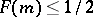and. Every random variable has at least one median. Iffor allin a closed interval, then every point of this interval is a median. Ifis a strictly-monotone function, then the median is unique. In the symmetric case, if the median is unique, it is identical with the mathematical expectation, provided that the latter exists. The fact that a median always exists is used for centering random variables (see, for instance, Lévy inequality). In mathematical statistics, to estimate the median of a distribution in terms of independent results of observationsone uses a so-called sample median — a median of the corresponding order statistics (cf. Order statistic)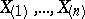, that is, of the quantity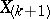if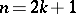is odd, and of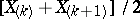if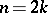is even.